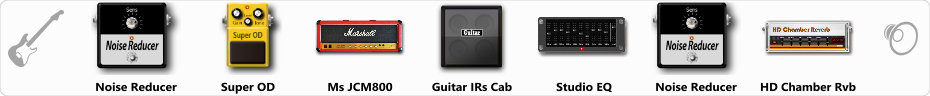# Ultimate live JCM800 [ovrdrvn]

Discussion in 'ToneLib-GFX presets' started by Jean C.Guion, Sep 23, 2019.

1. Ultimate live JCM800 [ovrdrvn]

Preset name: Ultimate live JCM800Live[ovrdrvn]

middle of the road everywhere ...Usable for any Marshall sound...not too much of anything..

Effects chain:Effect: "Noise Reducer" (Dynamics / Filter), active - "yes"
"Sens" = 32
"Mode" = Hard

Effect: "Super OD" (Overdrive / Distortion), active - "yes"
"Drive" = 54
"Tone" = 20
"Level" = 33

Effect: "Ms JCM800" (Amp simulators), active - "yes"
"Gain" = 84
"Bass" = 84
"Middle" = 75
"Treble" = 76
"Presence" = 17
"Master" = 77
"Output" = 50
"Level (dB)" = -2

Effect: "Guitar IRs Cab" (Cabinets), active - "yes"
"Model" = Marshall 1960A (4x12")
"Mic Position" = Edge
"Mic Distance" = Far
"Low Cut (Hz)" = 76
"Hi Cut (kHz)" = 7.5
"Mix" = 100
"Level (dB)" = 3

Effect: "Studio EQ" (Dynamics / Filter), active - "yes"
"31 Hz" = -8
"62 Hz" = 5
"125 Hz" = 6
"250 Hz" = 0
"500 Hz" = 2
"1 kHz" = 1
"2 kHz" = 9
"4 kHz" = -13
"8 kHz" = -15
"16 kHz" = -15
"above 16 kHz" = 0
"Level (dB)" = -1

Effect: "Noise Reducer" (Dynamics / Filter), active - "yes"
"Sens" = 29
"Mode" = Hard

Effect: "HD Chamber Rvb" (Reverb), active - "yes"
"Time" = 8.2
"PreLPF" = 85
"PreDelay" = 56
"HiDamp" = 23
"LoGain" = -3.1
"Mix" = 40

Note: You will need to download and install the ToneLib-GFX software to use the preset.

File size:
651 bytes
Views:
3,196